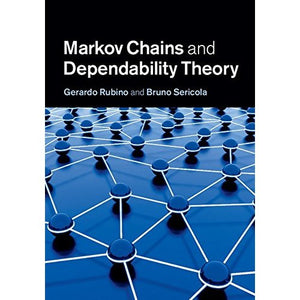># Markov Chains and Dependability Theory

• £4.59
• Save £60

Gerardo Rubino, Bruno Sericola
Cambridge University Press, 6/12/2014
EAN 9781107007574, ISBN10: 1107007577

Hardcover, 284 pages, 24.7 x 17.4 x 1.8 cm
Language: English

Dependability metrics are omnipresent in every engineering field, from simple ones through to more complex measures combining performance and dependability aspects of systems. This book presents the mathematical basis of the analysis of these metrics in the most used framework, Markov models, describing both basic results and specialised techniques. The authors first present both discrete and continuous time Markov chains before focusing on dependability measures, which necessitate the study of Markov chains on a subset of states representing different user satisfaction levels for the modelled system. Topics covered include Markovian state lumping, analysis of sojourns on subset of states of Markov chains, analysis of most dependability metrics, fundamentals of performability analysis, and bounding and simulation techniques designed to evaluate dependability measures. The book is of interest to graduate students and researchers in all areas of engineering where the concepts of lifetime, repair duration, availability, reliability and risk are important.

1. Introduction
2. Discrete time Markov chains
3. Continuous time Markov chains
4. State aggregation of Markov chains
5. Sojourn times in subsets of states
6. Occupation times
7. Performability
8. Stationary detection
9. Simulation of dependability models
10. Bounding techniques.